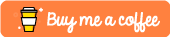Implementing Quicksort in Python

This tutorial was built using Python 3.6

Quicksort is one of the most popular sorting algorithms you will come across in computer science. This is because of it’s average case performance of O(n log n).

The Quicksorting algorithm uses a divide-and-conquer approach and recursively partitions the elements in an unsorted array around a randomly picked pivot element.

• A Random Pivot element is chosen from our unsorted array.
• We create 3 distinct partitions:
• Equal - for all elements equal to our pivot element
• Lower - for all elements lower than our pivot element
• Higher - for all elements higher than our pivot element
• We iterate through our array and sort those that are less than our pivot into the lower partition
• We sort those that are higher than our pivot into the higher partition
• We then recursively sort through these higher and lower partitions

Whilst this typically runs with a performance of O(n log n), it should be noted that the worst case performance for this algorithm is actually O(N^2) which occurs if you consistently choose the worst possible point in the array to pivot on.

Implementing this in Python

Now that we understand the logic behind the quicksorting algorithm, it’s time to implement this in Python. Below we’ll be defining a quicksort() function which will take in an array as it’s only parameter.

def quicksort(array):

# We define our 3 arrays
less = []
equal = []
greater = []

# if the length of our array is greater than 1
# we perform a sort
if len(array) > 1:
# Select our pivot. This doesn't have to be
# the first element of our array
pivot = array

# recursively go through every element
# of the array passed in and sort appropriately
for x in array:
if x < pivot:
less.append(x)
if x == pivot:
equal.append(x)
if x > pivot:
greater.append(x)

# recursively call quicksort on gradually smaller and smaller
# arrays until we have a sorted list.
return quicksort(less)+equal+quicksort(greater)

else:
return array

Testing it works

We can test this by doing the following:

>>> import quickSort
>>> quickSort.quicksort([6,4,7,1,2,9,12,3])
[1, 2, 3, 4, 6, 7, 9, 12]

Conclusion

If you found this tutorial useful or require further assistance or info then please let me know in the comments section below!